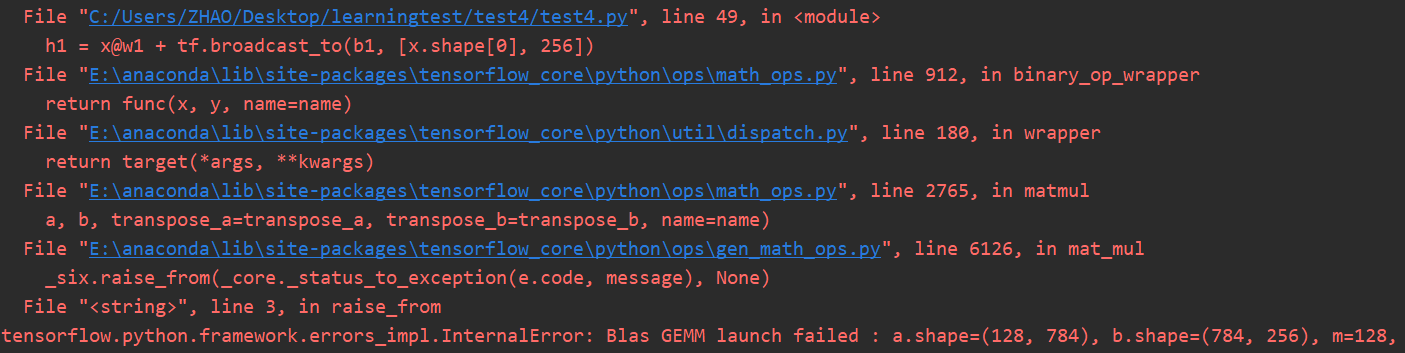tensorflow.python.framework.errors_impl.InternalError: Blas GEMM launch failed ，程序中出现anaconda错误？``````import  tensorflow as tf
from    tensorflow import keras
from    tensorflow.keras import datasets
import  os

os.environ['TF_CPP_MIN_LOG_LEVEL'] = '2'

# x: [60k, 28, 28],
# y: [60k]
# x: [0~255] => [0~1.]
x = tf.convert_to_tensor(x, dtype=tf.float32) / 255.
y = tf.convert_to_tensor(y, dtype=tf.int32)

print(x.shape, y.shape, x.dtype, y.dtype)
print(tf.reduce_min(x), tf.reduce_max(x))
print(tf.reduce_min(y), tf.reduce_max(y))

train_db = tf.data.Dataset.from_tensor_slices((x,y)).batch(128)
train_iter = iter(train_db)
sample = next(train_iter)
print('batch:', sample.shape, sample.shape)

# [b, 784] => [b, 256] => [b, 128] => [b, 10]
# [dim_in, dim_out], [dim_out]
w1 = tf.Variable(tf.random.truncated_normal([784, 256], stddev=0.1))
b1 = tf.Variable(tf.zeros())
w2 = tf.Variable(tf.random.truncated_normal([256, 128], stddev=0.1))
b2 = tf.Variable(tf.zeros())
w3 = tf.Variable(tf.random.truncated_normal([128, 10], stddev=0.1))
b3 = tf.Variable(tf.zeros())

lr = 1e-3

for epoch in range(10): # iterate db for 10
for step, (x, y) in enumerate(train_db): # for every batch
# x:[128, 28, 28]
# y: 

# [b, 28, 28] => [b, 28*28]
x = tf.reshape(x, [-1, 28*28])

with tf.GradientTape() as tape: # tf.Variable
# x: [b, 28*28]
# h1 = x@w1 + b1
# [b, 784]@[784, 256] +  => [b, 256] +  => [b, 256] + [b, 256]
h1 = x@w1 + tf.broadcast_to(b1, [x.shape, 256])
h1 = tf.nn.relu(h1)
# [b, 256] => [b, 128]
h2 = h1@w2 + b2
h2 = tf.nn.relu(h2)
# [b, 128] => [b, 10]
out = h2@w3 + b3

# compute loss
# out: [b, 10]
# y: [b] => [b, 10]
y_onehot = tf.one_hot(y, depth=10)

# mse = mean(sum(y-out)^2)
# [b, 10]
loss = tf.square(y_onehot - out)
# mean: scalar
loss = tf.reduce_mean(loss)

# w1 = w1 - lr * w1_grad